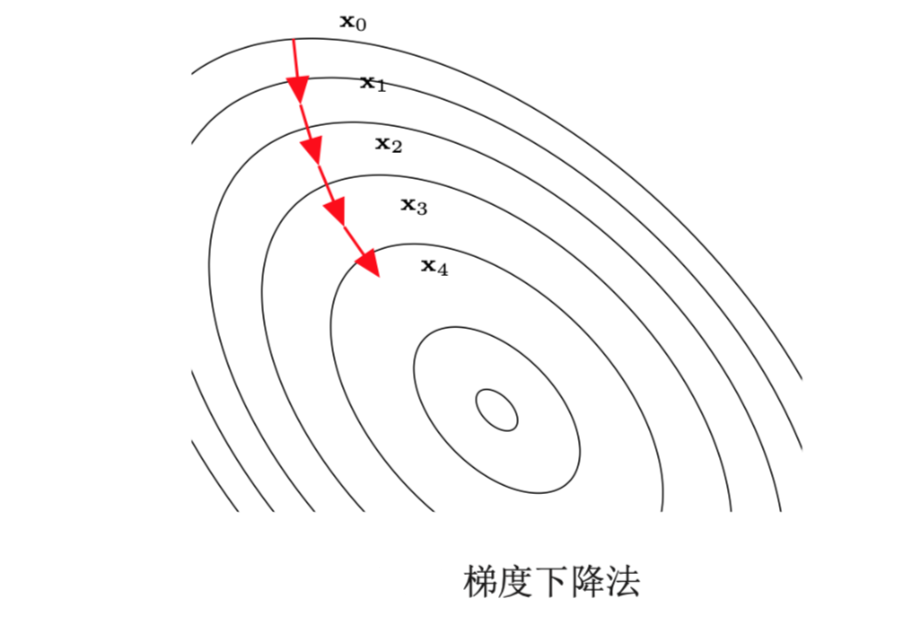【算法工程师的数学基础】系列将会从线性代数、微积分、数值优化、概率论、信息论五个方面进行介绍，感兴趣的欢迎关注【搜索与推荐Wiki】公众号，获得最新文章。

《算法工程师的数学基础》已更新：

# 数学优化

## 数学优化的类型

### 离散优化和连续优化

#### 离散优化问题

• 1、组合优化（Combinational Optimization）：其目标是从一个有限集合中找出使得目标函数最优的元素。在一般的组合优化问题中，集合中的元素之间存在一定的关联，可以表示为图结构。典型的组合优化问题有旅行商问题、最小生成树问题、图着色问题等。很多机器学习问题都是组合优化问题，比如特征选择、聚类问题、超参数优化问题以及结构化学习（Structuced Learning）中标签预测问题等。
• 2、整数规划（Integer Programming）：输入变量$x \in Z^d$为整数。一般常见的整数规划问题为整数线性规划（Integer Linear Programming， ILP）。整数线性规划的一种最直接的求解方法是：

• 1）去掉输入为整数的限制，得到一个就称为一个一般的线性规划问题，这个线性规划问题为原整数线性规划问题的松弛问题；
• 2）求得相应松弛问题的解；
• 3）把松弛问题的解四舍五入到最接近的整数。但是这种方法得到的解一般都不是最优的，因此原问题的最优解不一定在松弛问题最优解的附近

另外，这种方法得到的解也不一样定满足约束条件。

## 优化算法

### 梯度下降法## 拉格朗日乘数法与KKT条件

1、无约束条件

1. 等式约束条件

设目标函数是$f(x)$，等式约束函数是$g(x)$，即在$g(x)$满足的情况下求解$f(x)$的极值。常用的解决办法就是拉格朗日乘数法

2. 不等式约束条件

设目标函数是$f(x)$，不等式约束函数是$g(x)$，即在$g(x)$满足的情况下求解$f(x)$的极值。常用的解决办法就是KKT（Karush Kuhn Tucker）条件

### 不等式约束优化问题

• $a = [a_i, …, a_m]^T$为等式约束的拉格朗日乘数
• $b = [b_i, …, b_n]^T$为不等式约束的拉格朗日乘数

$\Gamma(a,b)$是一个凹函数，即使$f(x)$是非凸的。

KKT条件中需要关注的是$b^_j g_j (x^) =0$，称为互补松弛条件。如果最优解$x^$出现在不等式约束的边界上$g_j(x) = 0$，则$b^_j>0$；如果$x^$出现在不等式约束的内部$g_j(x) < 0$，则$b^_j=0$。互补松弛条件说明当最优解出现在不等式约束的内部，则约束失效。

【技术服务】，详情点击查看： https://mp.weixin.qq.com/s/PtX9ukKRBmazAWARprGIAg